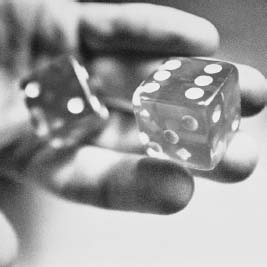NextPrevious

# What is the Markov model?

The Markov model was first developed by Russian mathematician Andrey (Andrei) Andreyevich Markov (1856–1922) about a century ago. It is a statistical method that estimates the likelihood of a future event based on patterns observed in the past. They are most useful when a decision problem involves risk that is continuous over time, when the timing of events is important, and when important events may happen more than once.

The Markov chain also has applications in statistical models, and is a mathematical system that undergoes transitions from one state to another in a chainlike manner.

Most often today, it is used in such fields as economics, genetics, and computer speech-recognition. It is often used extensively in clinical medical studies; for example, Markov models assume that a patient is always in one of a finite number of discrete health states, called Markov states. Such studies can be evaluated by matrix algebra or as a Monte Carlo simulation.Games such as coin tossing and dice rolling are common examples of the rules of probability in action.

Close

This is a web preview of the "The Handy Math Answer Book" app. Many features only work on your mobile device. If you like what you see, we hope you will consider buying. Get the App